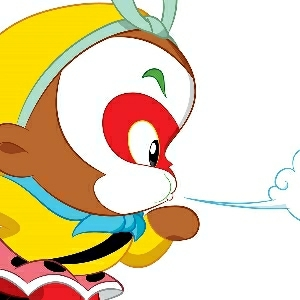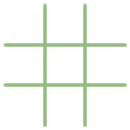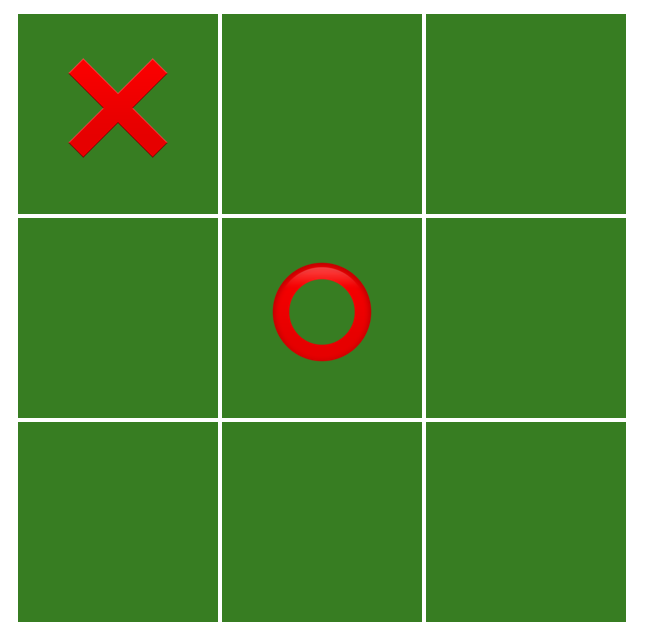# 编程能力 —— TicTacToe（井字棋）wendraw# 1. 画棋盘

<style></style><div id="board"></div><script>  let pattern = [    2, 0, 0,    0, 1, 0,    0, 0, 0  ];  function show(pattern) {    let board = document.getElementById('board');    board.innerHTML = "";    for (let y = 0; y < 3; y++) {      for (let x = 0; x < 3; x++) {        let cell = document.createElement('div');        cell.classList.add("cell")        cell.innerHTML = pattern[y * 3 + x] === 2 ? "❌" :             pattern[y * 3 + x] === 1 ? "⭕️" : "";        board.appendChild(cell);      }      board.appendChild(document.createElement("br"));    }  }  show(pattern);</script>image.png

# 2. 添加落子

<script>  let color = 1;  function show(pattern) {    // ......    cell.innerHTML = pattern[y * 3 + x] === 2 ? "❌" :        pattern[y * 3 + x] === 1 ? "⭕️" : "";    cell.addEventListener('click', () => move(x, y));    board.appendChild(cell);    // ......  }  function move(x, y) {    if (pattern[y * 3 + x] > 0) return;        pattern[y * + x] = 3 - color;    color = 3 - color;    show(pattern);  }</script>

# 3. 判断输赢

<script>  // ......  function check(pattern, color) {    for (let y = 0; y < 3; y++) {      let win = true;      for (let x = 0; x < 3; x++) {        if (pattern[y * 3 + x] !== color) {          win = false;          break;        }      }      if (win) return true;    }    for (let y = 0; y < 3; y++) {      let win = true;      for (let x = 0; x < 3; x++) {        if (pattern[x * 3 + y] !== color) {          win = false;          break;        }      }      if (win) return true;    }    {   // 正对角线      let win = true;      for (let i = 0; i < 3; i++) {        if (pattern[i * 3 + i] !== color) {          win = false;          break;        }      }      if (win) return true;    }    {   // 反对角线      let win = true;      for (let i = 0; i < 3; i++) {        if (pattern[i * 3 + 2 - i] !== color) {          win = false;          break;        }      }      if (win) return true;    }    return false;  }</script>

## Best choice

function bestChoice(pattern, color) {  // 判断自己是否能赢  let point = willWin(pattern, color);  if (point) {    return {      point: point,      result: 1,    }  }  let result = -1;  for (let y = 0; y < 3; y++) {    for (let x = 0; x < 3; x++) {      // 遍历所有空位，找到对对方最不利的局面      if (pattern[y * 3 + x] !== 0) continue;      let tmp = clone(pattern);      tmp[y * 3 + x] = color;      let opp = bestChoice(tmp, 3 - color); // 对方的最佳选择      // 选择对方最差的结果，并比我们当前的更好结果，作为我们的选择      if (-opp.result >= result) {        point = [x, y];        result = -opp.result;      }    }  }  return {    point: point,    result: point ? result : 0,  }}

## 剪枝

outer:for (let y = 0; y < 3; y++) {  for (let x = 0; x < 3; x++) {    // 遍历所有空位，找到对对方最不利的局面    if (pattern[y * 3 + x] !== 0) continue;    let tmp = clone(pattern);    tmp[y * 3 + x] = color;    let opp = bestChoice(tmp, 3 - color); // 对方的最佳选择    // 选择对方最差的结果，并比我们当前的更好结果，作为我们的选择    if (-opp.result >= result) {      point = [x, y];      result = -opp.result;    }    // 利用 JS 中的 label 标签来跳出外层循环    if (result === 1) break outer;  }}

## computerMove 和 userMove

computerMove 函数的逻辑跟 userMove 一样，只不是坐标 x、y 是由 bestChoice 给我们生成。

function userMove(x, y) {  if (pattern[y * 3 + x] > 0) return;  pattern[y * 3 + x] = color;  show(pattern);  if (check(pattern, color)) {    alert(${color === 2 ? "❌" : "⭕️"} is winner); } color = 3 - color; computerMove();}function computerMove() { let choice = bestChoice(pattern, color); if (choice.point) { pattern[choice.point * 3 + choice.point] = color; } show(pattern); if (check(pattern, color)) { alert(${color === 2 ? "❌" : "⭕️"} is winner);  }  color = 3 - color;}

## 棋谱

const openings = new Map();openings.set(  [0, 0, 0, 0, 0, 0, 0, 0, 0].toString() + "1",  { point: [1, 1], result: 0 });openings.set(  [0, 0, 0, 0, 1, 0, 0, 0, 0].toString() + "2",  { point: [0, 0], result: 0 });function bestChoice(pattern, color) {  if (openings.has(pattern.toString() + color)) {    return openings.get(pattern.toString() + color);  }  // ......}

# 参考

winter 前端进阶训练营## 评论